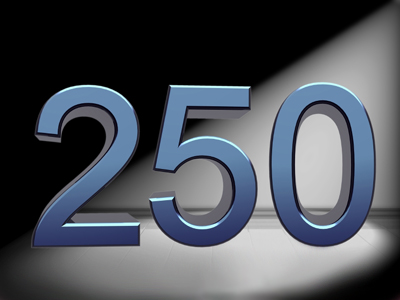250 is a multiple of 50.

# Multiples 1

This Math quiz is called 'Multiples 1' and it has been written by teachers to help you if you are studying the subject at elementary school. Playing educational quizzes is an enjoyable way to learn if you are in the 3rd, 4th or 5th grade - aged 8 to 11.

It costs only \$12.50 per month to play this quiz and over 3,500 others that help you with your school work. You can subscribe on the page at Join Us

A multiple of a number is the result of multiplication with some other whole number, for example 3 x 5 = 15 so 15 is a multiple of 3 and 5. Knowing the times tables for different numbers will help you to work out multiples. Each of the numbers in a times table is a multiple of the first number, for example 5, 10, 15, 20, 25 and 30 are all multiples of 5. The same rule applies in all of the times tables.

How much have you remembered from your school classes about multiples? Take this quiz for 7-8 year olds all about multiples and multiplication and find out!

1.
Which is the next multiple of 5 after 120?
115
121
125
130
2.
What is the next multiple of 2 after 198?
199
200
202
208
198 + 2 = 200
3.
Which of these is a multiple of 50?
75
220
250
205
A multiple of 50 always ends in 00 or 50
4.
Which is a multiple of 50 and 100?
50
200
250
750
As 100 is a multiple of 50, all multiples of 100 are also multiples of 50
5.
A multiple of 5 always ends in?
0 or 2
0
0 or 00
0 or 5
Think of the numbers in the 5 times table: 5, 10, 15, 20, 25, 30 etc
6.
Which is the multiple of 100 before 1,000?
100
500
900
950
1,000 - 100 = 900
7.
Which of these is a multiple of 2 and 5?
2
5
10
15
2 x 5 = 10.
20, 30 and all other multiples of 10 are also multiples of 2 and 5
8.
Which is the smallest multiple of 100?
50
100
200
300
Every number is a multiple of itself as it is itself multiplied by 1
9.
A multiple of 2 is always?
An odd number
A multiple of 5
An even number
A number ending in 2
A multiple of 2 ends in 0, 2, 4, 6 or 8 and is therefore an even number
10.
What does a multiple of 100 always end in?
00
2
5
11
Similarly, multiples of 10 (20, 30, 40 etc.) always end in 0
Author:  Amanda Swift